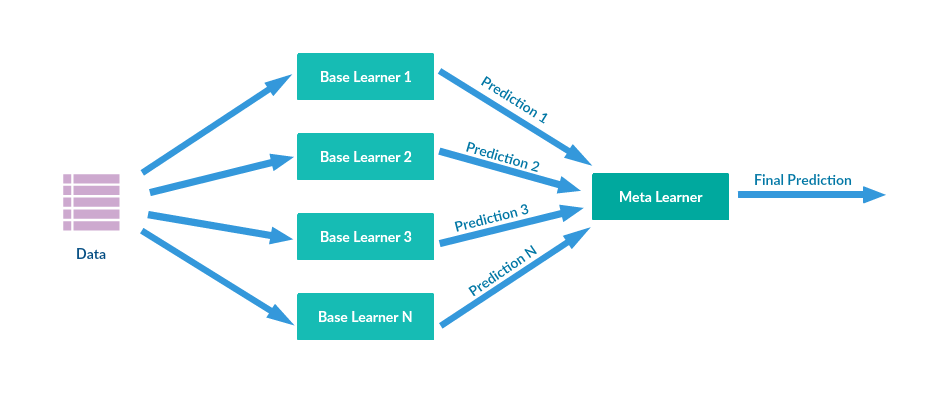# Pick n Mix¶A simple tool to help building stacking models.

## Features¶

Pick n Mix is a simple stacking tool for stacking Sci-Kit learn models of your picks. It provided 2 classes: Layer and Stack. Layer is a parallel combination of models, while Stack combine Layers to create a stacking model.

## Stacking¶

It’s one technique to ensemble (combine) multiple models into one more effective model by increasing the predictive force of the classifier. The idea is to train several models, each with the same objective but with different features or architectures.

Most of the errors from a model’s learning are from three main factors: variance, noise, and bias. By using ensemble methods, we’re able to increase the stability of the final model and reduce the errors mentioned previously. While they are individually not great, the main principle behind ensemble modeling is to group weak learners together to form one strong learner.A stacking new model (meta learner) is trained from the combined predictions of two (or more) previous model. The predictions from the models are used as inputs for each sequential layer, and combined to form a new set of predictions. These can be used on additional layers, or the process can stop here with a final result. Constant adding more layers just don’t lineally mean a better predictor.

## How to Install¶

### Stable Release¶

To install Pick n Mix, run this command in your terminal:

```\$ pip install picknmix
```

This is the preferred method to install Pick n Mix, as it will always install the most recent stable release.

If you don’t have pip installed, this Python installation guide can guide you through the process.

### From sources¶

• You can either clone the public repository:

```\$ git clone git://github.com/picknmix/picknmix
```
```\$ curl  -OL https://github.com/picknmix/picknmix/tarball/master
```
• Once you have a copy of the source, you can install it with:

```\$ python -m pip install .
```

## Usage¶

Use Pick n Mix to create a regression model:

```from picknmix import Layer, Stack

import numpy as np
from sklearn.linear_model import LinearRegression
from sklearn.linear_model import Ridge

first_layer = Layer([LinearRegression(), Ridge()])
second_layer = Layer([LinearRegression()])
model = Stack([first_layer, second_layer])

X = np.array([[1, 1], [1, 2], [2, 2], [2, 3]])
y = np.dot(X, np.array([1, 2])) + 3
model.fit(X, y)
model.predict(np.array([[3, 5]]))
```

You can also use preprocessing in a Layer:

```from sklearn.linear_model import MinMaxScaler

first_layer = Layer([LinearRegression(), Ridge()],
preprocessors = [MinMaxScaler(), None])
```

For more examples for usage, please refer to the documentation.